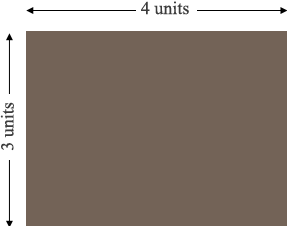SEARCH HOMEMath Central Quandaries & QueriesQuestion from John: What are the dimensions of a 12-acre parcel if it is 3 square acres deep and 4 square acres wide?Hi John,

I am not sure what you are asking but I am going to guess. If I have misinterpreted your question please write back.

I think you have a parcel of land with an area of 12 acres and the ratio of the width to the depth is 4 to 3.There are 43,560 square feet in an acre so the area of your land can be expressed as $12 \times 43560$ square feet. There is some unknown number $x$ so that your land is $4 \times x$ feet by $3 \times x$ feet. Since the area of a rectangle is its length times its width we have

$4 x \times 3 x = 12 \times 43560$

dividing both sides by 12 gives $x^2 = 43560$ or $x = \sqrt{43560} = 208.71$ feet. Thus your parcel of land is $4 \times 208.71$ feet by $3 \times 208.71$ feet.

PennyMath Central is supported by the University of Regina and The Pacific Institute for the Mathematical Sciences.